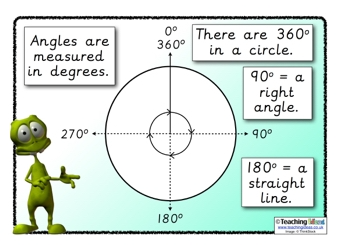# Maths

Right Angle = 90 degrees

Angles on a straight line = 180 degrees

Angles in a circle = 360 degrees

Angles in a Triangle = 180 degrees

Angles in a quadrilateral = 360 degreesWednesday

LO: To calculate missing angles on a point

Thursday

LO: To calculate missing angles in a triangle

Friday

LO: To calculate missing angles in a quadrilateral